## Pages

Atlanta Real Food is run by the Atlanta area chapter leaders of The Weston A. Price Foundation. Here you will find the latest news from local farmers, get information on how to properly prepare real foods, and stay up to date on local events.

## Tuesday, February 26, 2019

### Don't miss out!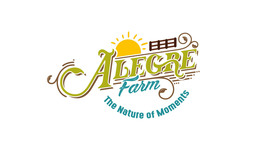## If you're only happy when the sun is out, you're missing half of your life. -Gloria Jones

 table div table+table+table+table div table{width:100%;padding:0}table div table+table+table+table div table img{width:96.23%;padding:0;float:none}table div table+table+table+table div table td{width:100%;padding:0 1.88% 18px}/* styles */## What you missed...

 /* styles */ So much has passed in a few short weeks. Here's some things you may have missed!
 table div table+table+table+table+table+table+table div table{width:100%;padding:0}table div table+table+table+table+table+table+table div table img{width:96.23%;padding:0;float:none}table div table+table+table+table+table+table+table div table td{width:100%;padding:0 1.88% 18px}/* styles */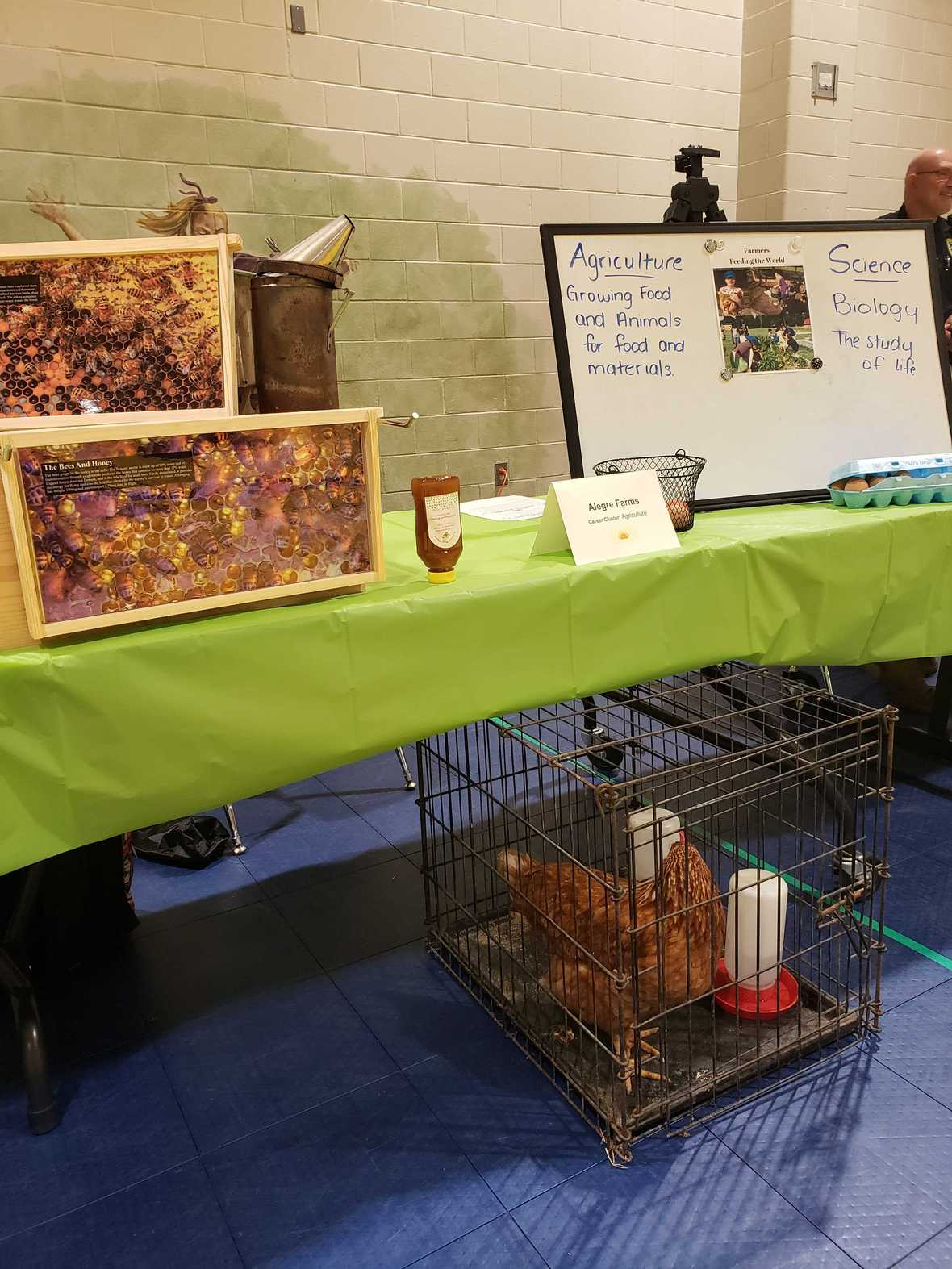Visiting Mulberry Elementary for Career Day
Last Friday, Alegre Farm answered students questions about agriculture. It helped them connect the dots to where eggs and chicken wings come from and understand that farmers have an important job: Feeding the world.

It clarified that as a farmer there are different specialties that one can focus on to produce different products: Cotton for clothes, bees for honey, cows for milk, and plants for veggies and fruits.

It's an important job and with many farmers beyond the age of 60, it's a career choice that we can't forget. Now with so much technology and engineering, farming has innovative practices.

 table div table+table+table+table+table+table+table+table+table div table{width:100%;padding:0}table div table+table+table+table+table+table+table+table+table div table img{width:96.23%;padding:0;float:none}table div table+table+table+table+table+table+table+table+table div table td{width:100%;padding:0 1.88% 18px}/* styles */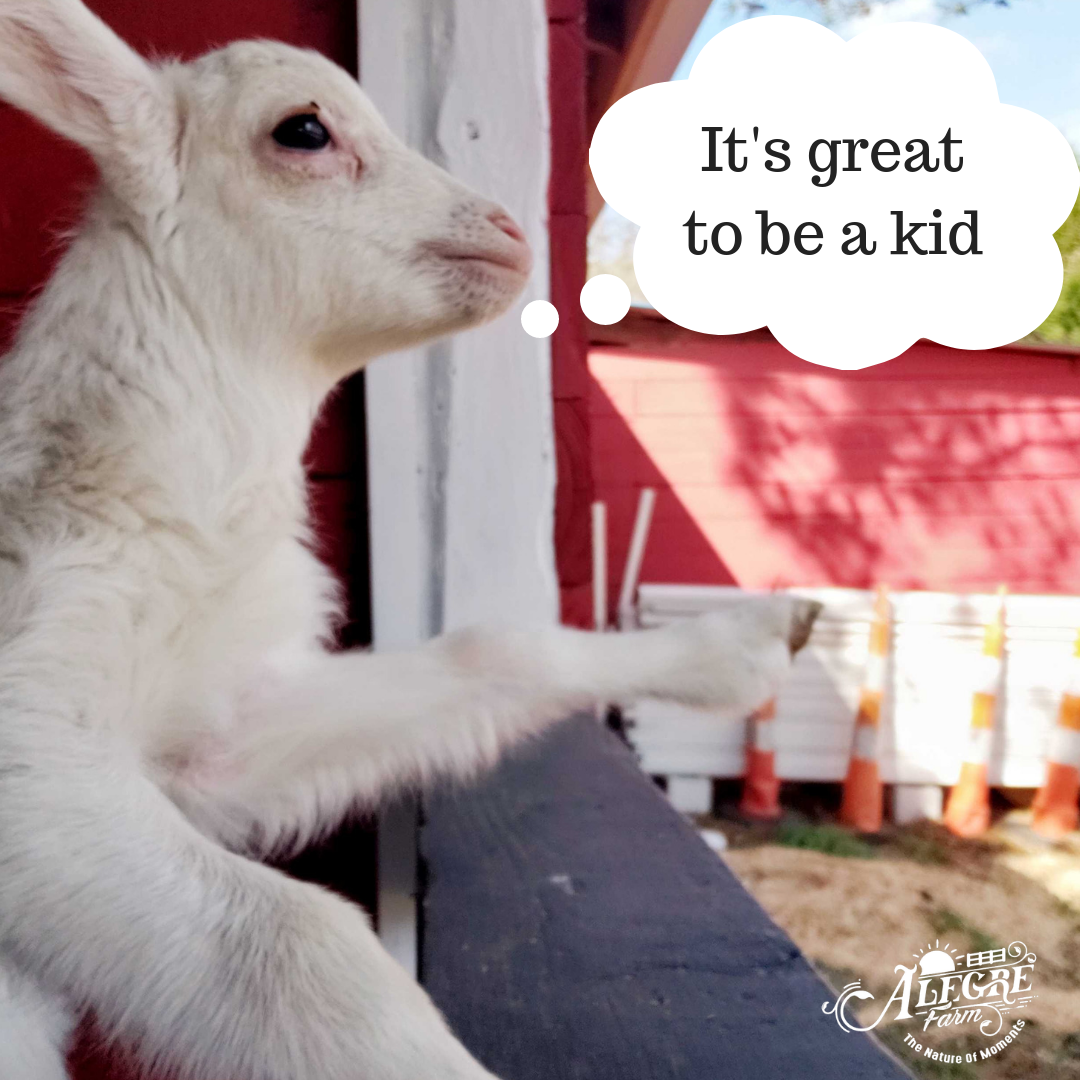Do you follow us on Facebook?
If not, you might want to...

Why? Because you're missing out on our contests!

Check out the latest winning post by Tara. Her comment of what Little Lamb was thinking had the most likes, so she won a prize!
What to know what she won? Then follow us on Facebook.

 table div table+table+table+table+table+table+table+table+table+table+table div table{width:100%;padding:0}table div table+table+table+table+table+table+table+table+table+table+table div table img{width:96.23%;padding:0;float:none}table div table+table+table+table+table+table+table+table+table+table+table div table td{width:100%;padding:0 1.88% 18px}/* styles */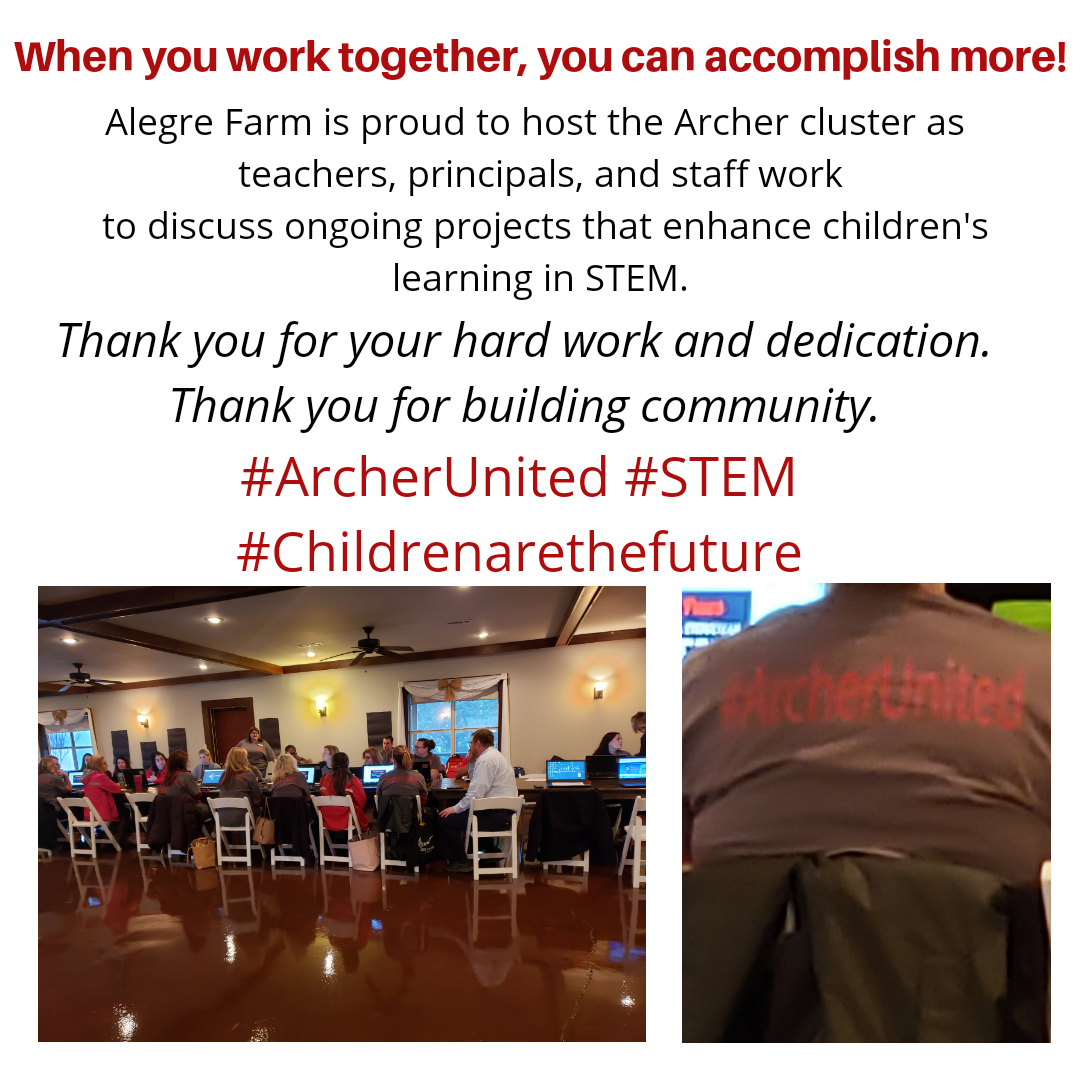The Archer Cluster is doing great things for their schools!

Archer Cluster has been working together from elementary to high school to ensure quality programs for students.

A large group of teachers and principals came to Alegre Farm to hold their day long meeting.

Even with the threatening weather, we were excited to give a tour of our education stations and give them a tractor ride!

Thank you for your dedication to your community!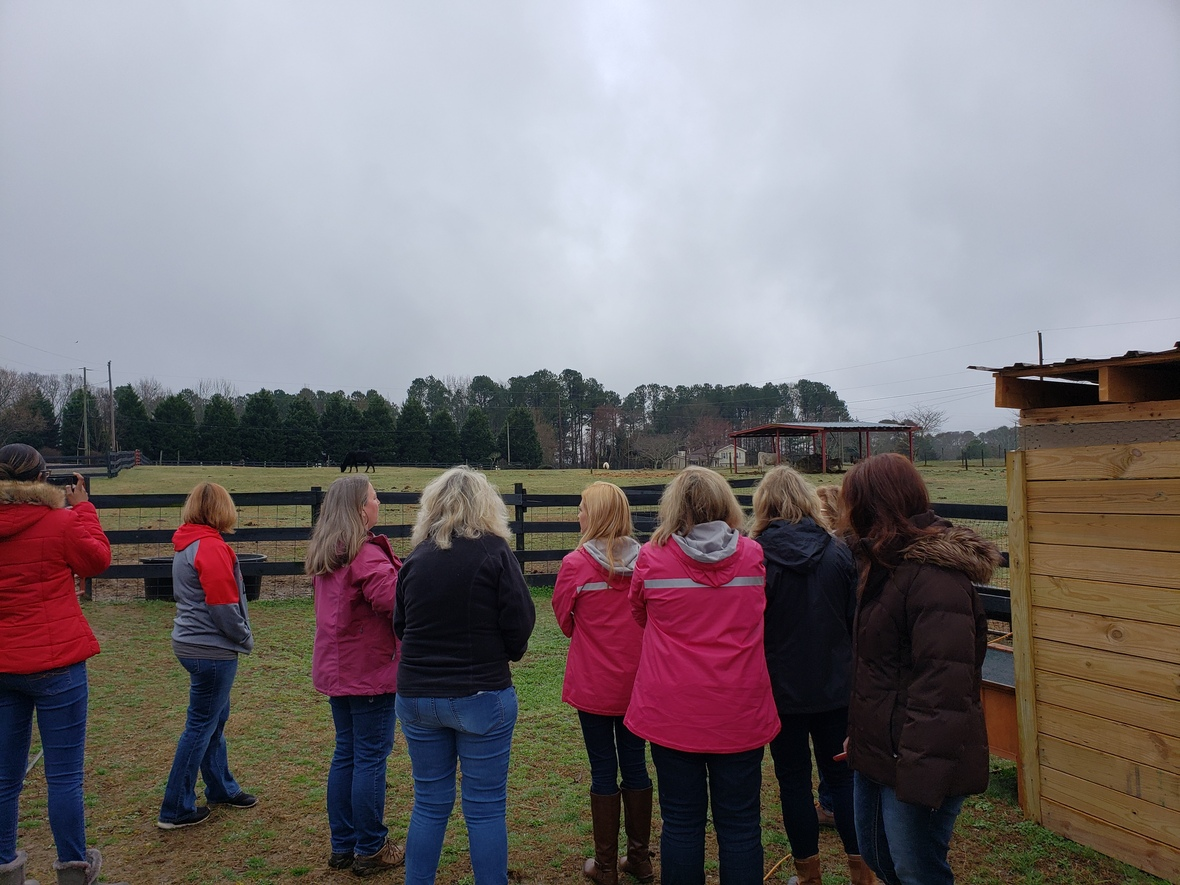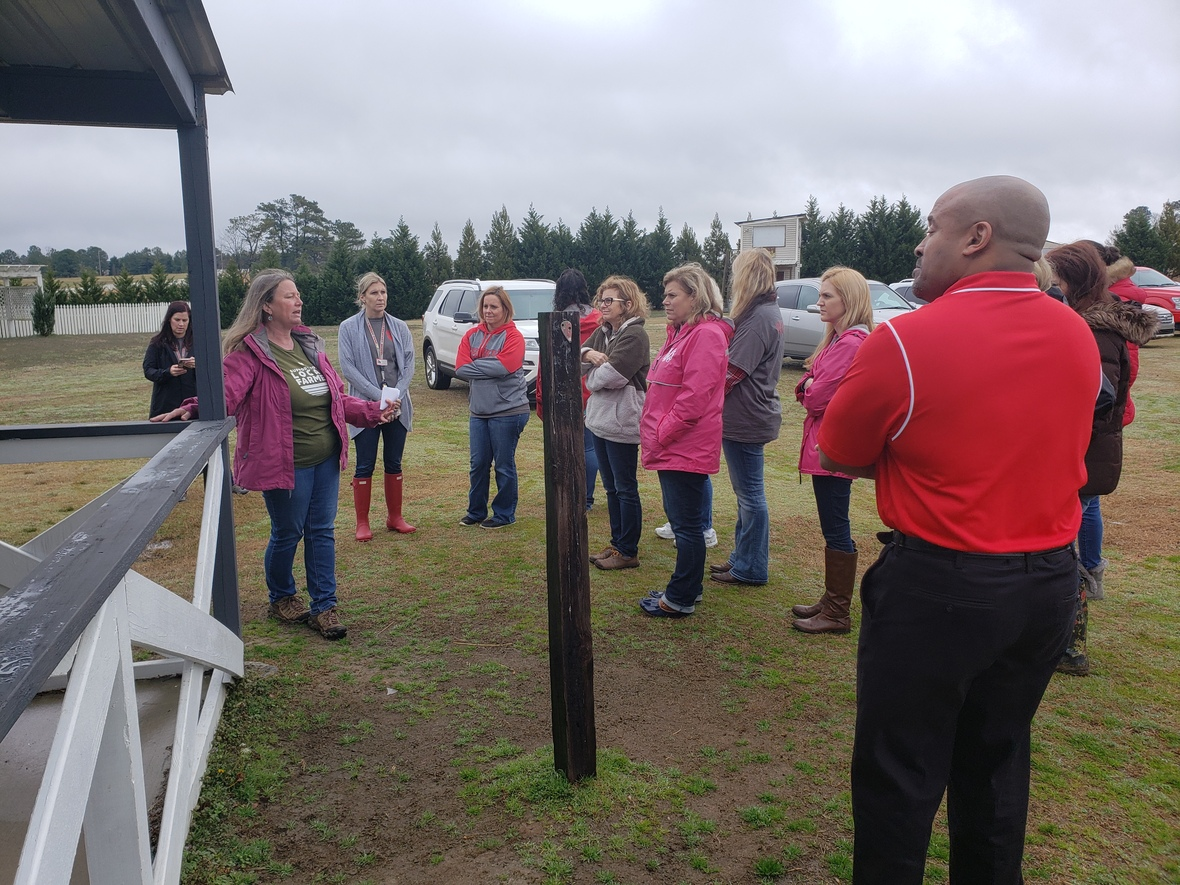table div table+table+table+table+table+table+table+table+table+table+table+table+table+table div table{width:100%;padding:0}table div table+table+table+table+table+table+table+table+table+table+table+table+table+table div table img{width:96.23%;padding:0;float:none}table div table+table+table+table+table+table+table+table+table+table+table+table+table+table div table td{width:100%;padding:0 1.88% 18px}/* styles *//* styles */ Always Growing, Always Learning Pilar has mentioned that she continuously considers new and different practices that can be brought to Alegre Farm and encourages staff to learn more about agriculture. On Saturday, a team member of Alegre Farm volunteered in South Atlanta and learned techniques on how to cultivate mushrooms! Sounds like another future prospect for Alegre Farm! We'll have to see...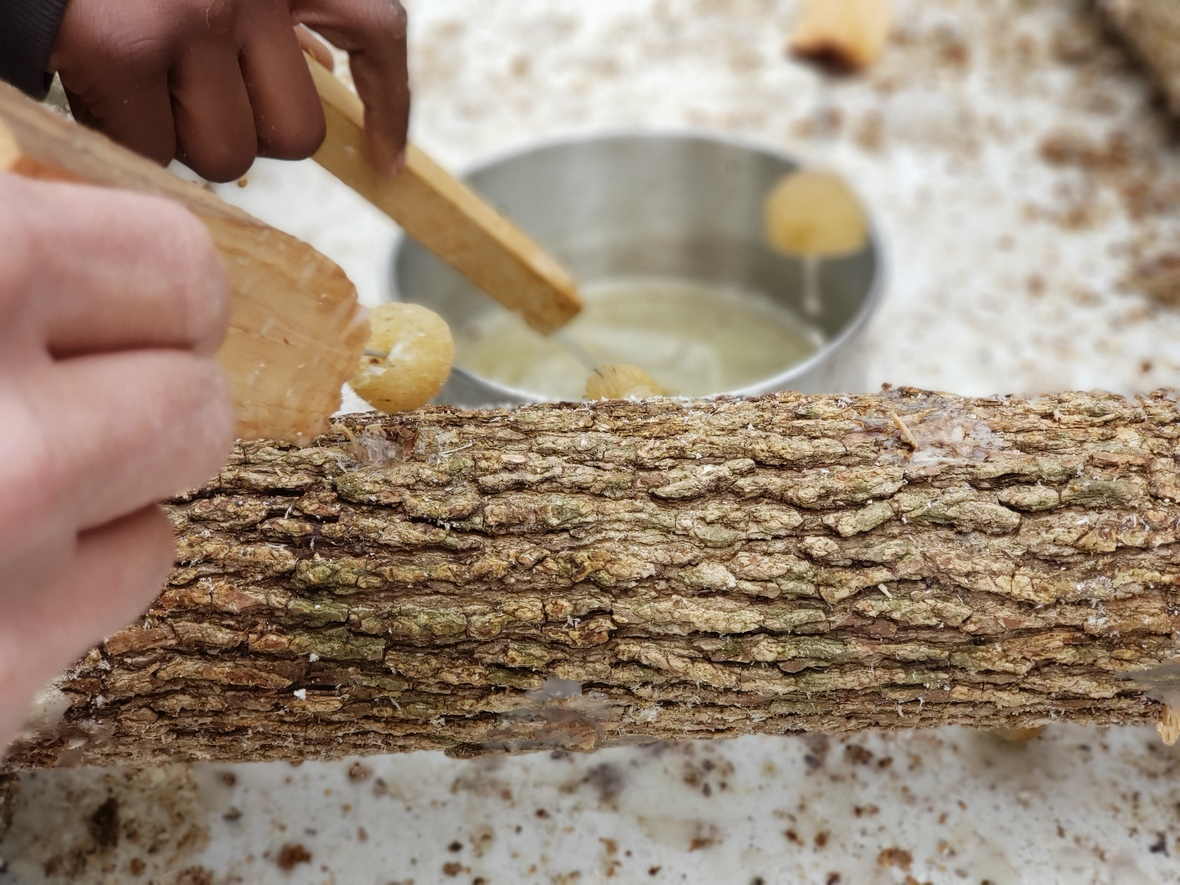Placing wax over the mushroom spores.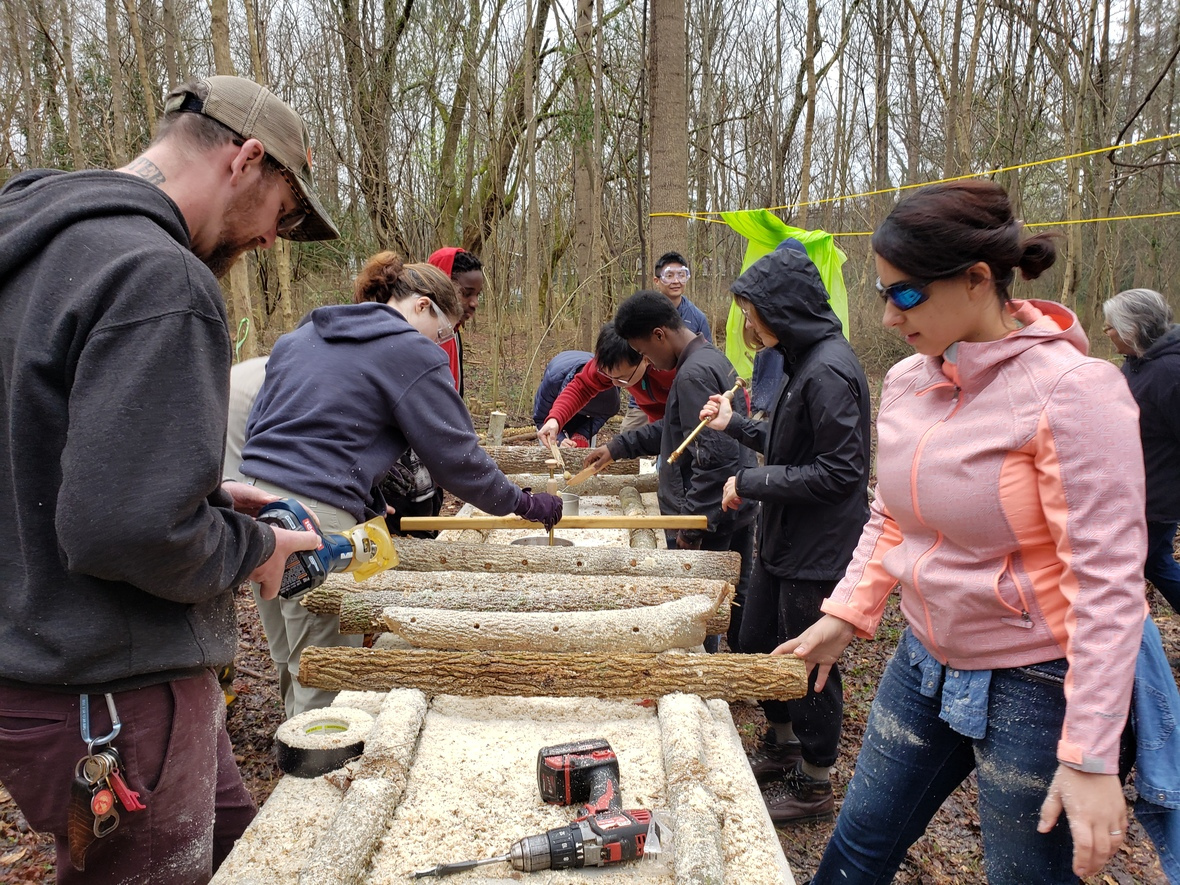Drilling holes in freshly cut sweet gum trees.
 table div table+table+table+table+table+table+table+table+table+table+table+table+table+table+table+table+table div table{width:100%;padding:0}table div table+table+table+table+table+table+table+table+table+table+table+table+table+table+table+table+table div table img{width:96.23%;padding:0;float:none}table div table+table+table+table+table+table+table+table+table+table+table+table+table+table+table+table+table div table td{width:100%;padding:0 1.88% 18px}/* styles */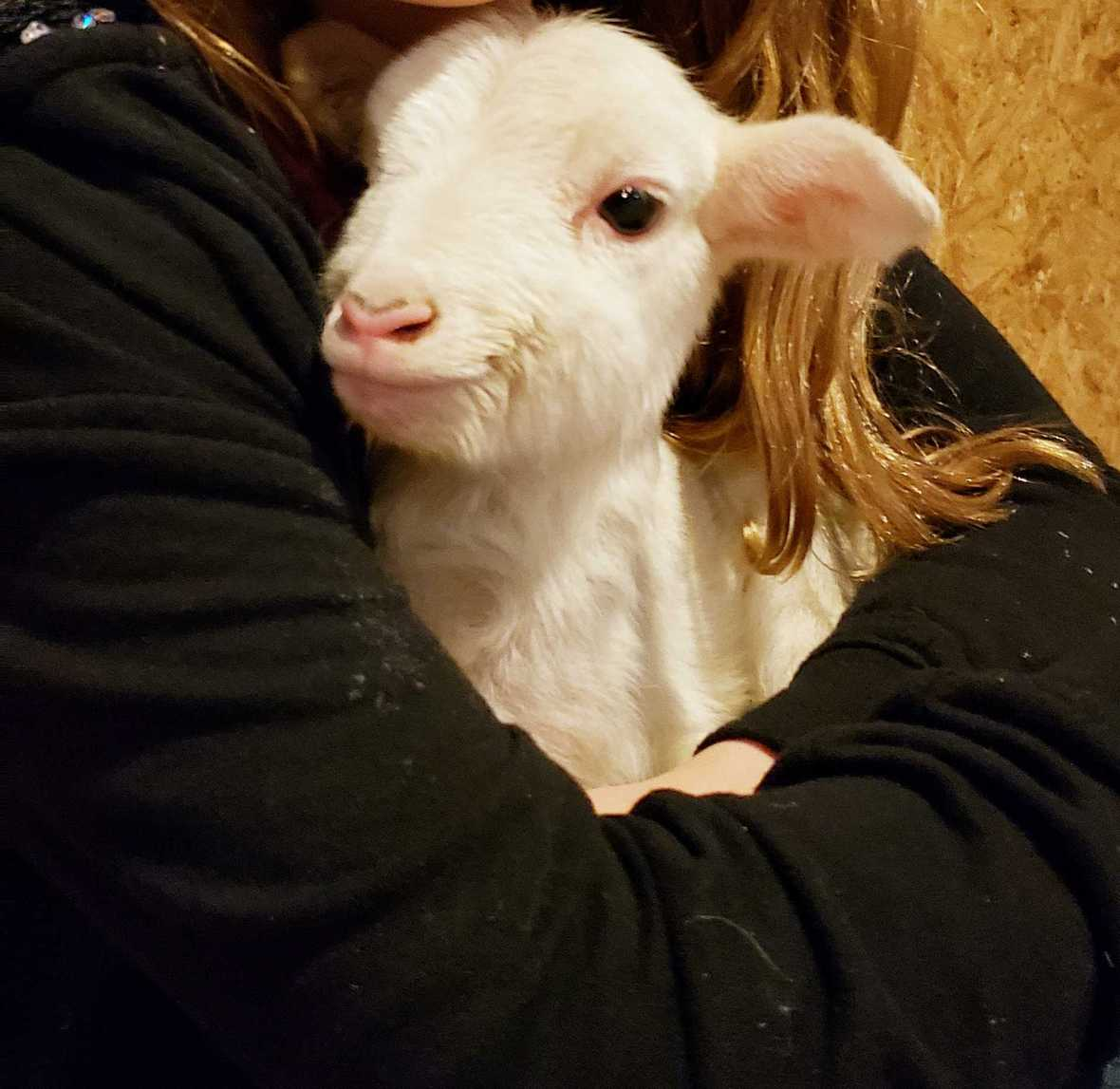Farm Camp was a hit!

We were so grateful for those who came to Presidents' Day Farm Camp. It was a day free of rain!

We had fun and enjoyed having your children embrace life on the farm. It was great to let them enjoy an animal encounter with our newly born lambs. Truly a unique experience.

Thank you for your support!

 table div table+table+table+table+table+table+table+table+table+table+table+table+table+table+table+table+table+table+table div table{width:100%;padding:0}table div table+table+table+table+table+table+table+table+table+table+table+table+table+table+table+table+table+table+table div table img{width:96.23%;padding:0;float:none}table div table+table+table+table+table+table+table+table+table+table+table+table+table+table+table+table+table+table+table div table td{width:100%;padding:0 1.88% 18px}/* styles */table div table+table+table+table+table+table+table+table+table+table+table+table+table+table+table+table+table+table+table+table+table div table{width:100%;padding:0}table div table+table+table+table+table+table+table+table+table+table+table+table+table+table+table+table+table+table+table+table+table div table img{width:96.23%;padding:0;float:none}table div table+table+table+table+table+table+table+table+table+table+table+table+table+table+table+table+table+table+table+table+table div table td{width:100%;padding:0 1.88% 18px}/* styles */## What you don't want to miss...

 table div table+table+table+table+table+table+table+table+table+table+table+table+table+table+table+table+table+table+table+table+table+table+table div table{width:100%;padding:0}table div table+table+table+table+table+table+table+table+table+table+table+table+table+table+table+table+table+table+table+table+table+table+table div table img{width:96.23%;padding:0;float:none}table div table+table+table+table+table+table+table+table+table+table+table+table+table+table+table+table+table+table+table+table+table+table+table div table td{width:100%;padding:0 1.88% 18px}/* styles */## 4-session Garden Workshop!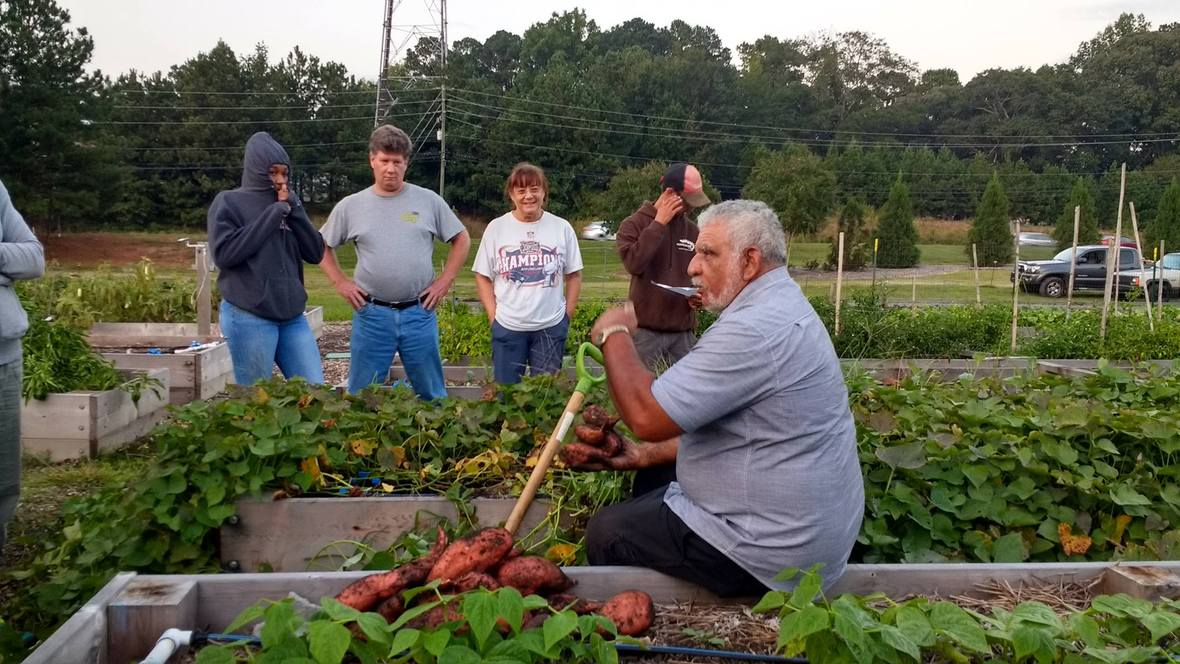Plan Your Spring Garden

For the first time, Alegre Farm will be bringing lead instructor of horticulture and 8th generation farmer, Tony Gobert, here for a garden workshop.

Tony will guide and show you the way to your best garden ever!

Whether you are experienced or new, these classes will help get you going further. Each class offers hands-on learning.

With only 15 seats available, don't wait to get your tickets for 4 sessions at only \$39.

 table div table+table+table+table+table+table+table+table+table+table+table+table+table+table+table+table+table+table+table+table+table+table+table+table+table+table+table div table{width:100%;padding:0}table div table+table+table+table+table+table+table+table+table+table+table+table+table+table+table+table+table+table+table+table+table+table+table+table+table+table+table div table img{width:96.23%;padding:0;float:none}table div table+table+table+table+table+table+table+table+table+table+table+table+table+table+table+table+table+table+table+table+table+table+table+table+table+table+table div table td{width:100%;padding:0 1.88% 18px}/* styles */## Farm Adventure Day Coming Soon!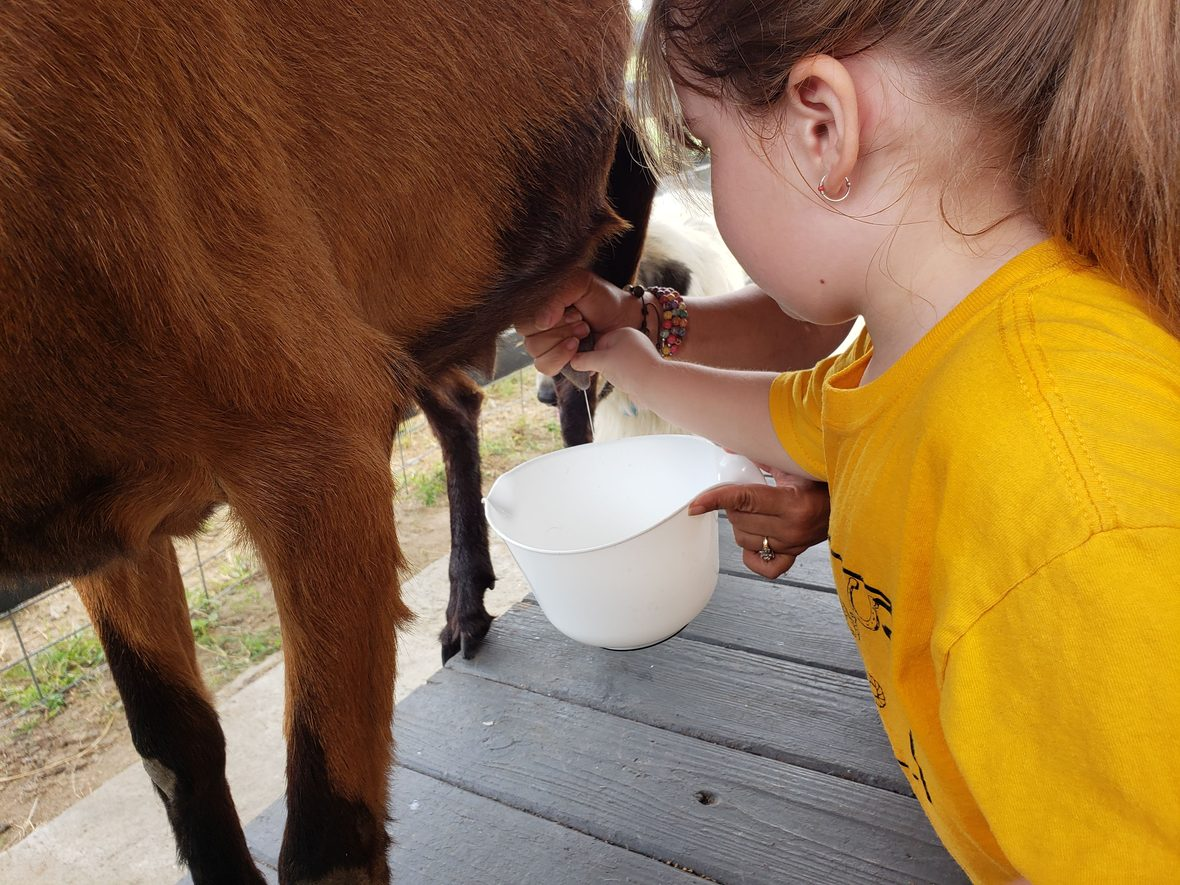# We anticipate that Farm Adventure Day will sell out.

10am-12pm on Friday, April 5th

Take a guided tour where you will meet new animals, smell the fresh flowers and herbs, learn how to make compost while the children have fun digging for worms, learn about our busy bees, go on a tractor ride!
Don't forget to bring your lunch to enjoy a picnic after the tour while your child can enjoy a pony ride!

Get Early Bird Pricing! Limited Tickets. We do not plan on having same day sales on event day.

 table div table+table+table+table+table+table+table+table+table+table+table+table+table+table+table+table+table+table+table+table+table+table+table+table+table+table+table+table+table+table+table+table div table{width:100%;padding:0}table div table+table+table+table+table+table+table+table+table+table+table+table+table+table+table+table+table+table+table+table+table+table+table+table+table+table+table+table+table+table+table+table div table img{width:96.23%;padding:0;float:none}table div table+table+table+table+table+table+table+table+table+table+table+table+table+table+table+table+table+table+table+table+table+table+table+table+table+table+table+table+table+table+table+table div table td{width:100%;padding:0 1.88% 18px}/* styles */## Help us choose our Q-Booch Label

 /* styles */ We have been working hard to expand our kombucha made here at Alegre Farm. We have transitioned on labels and want your help to choose our new label. Please vote on which color scheme you prefer! Also, if you know someone who may be interested in selling our satisfying kombucha at their location, please let us know at kombucha@alegrefarm.com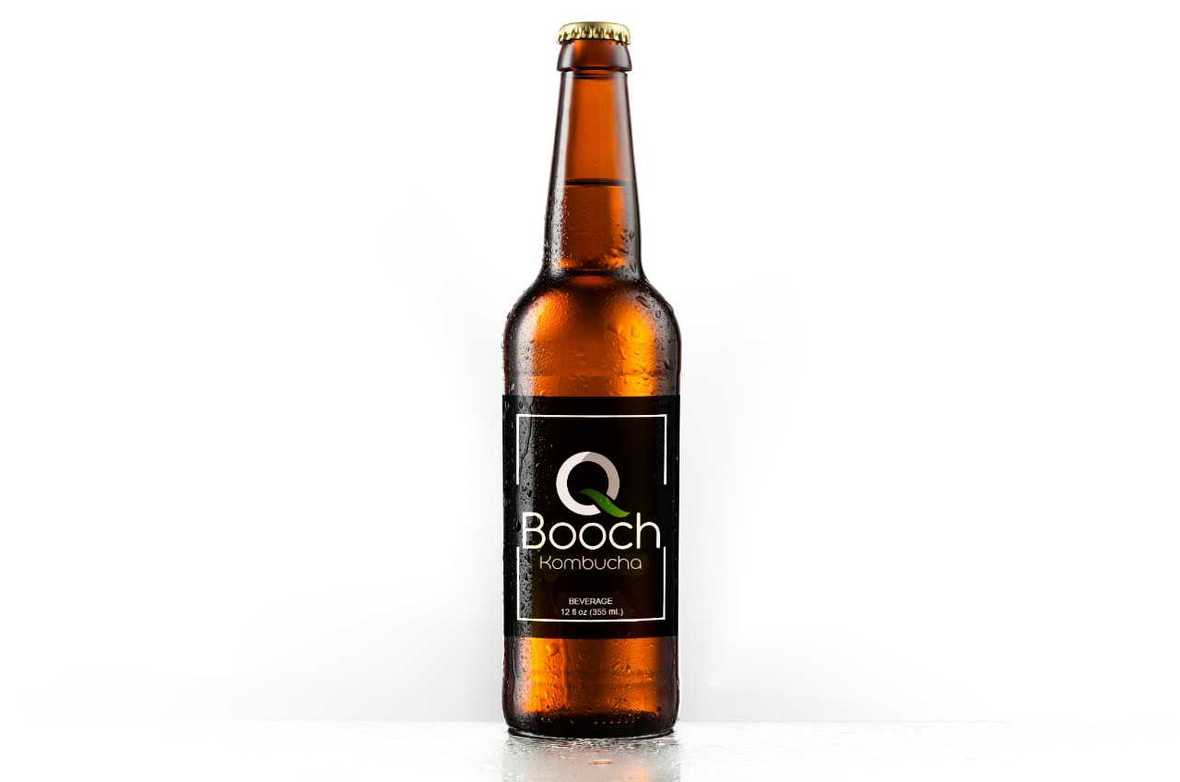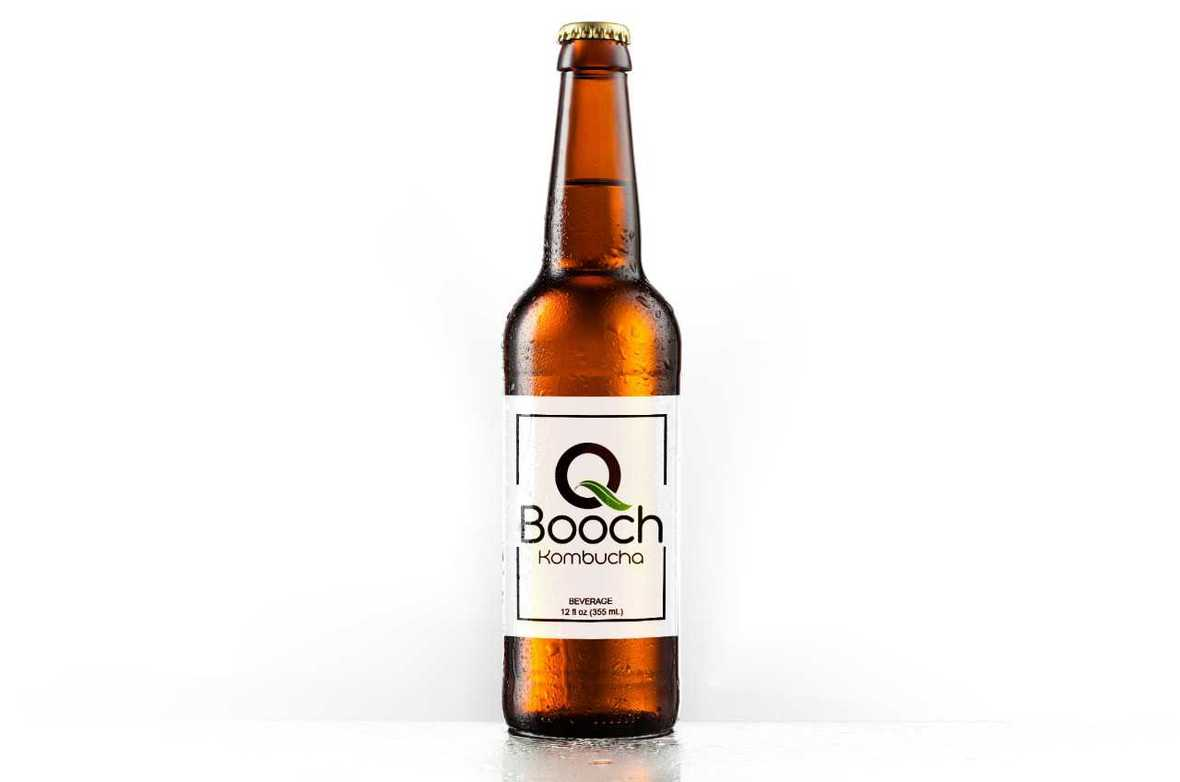table div table+table+table+table+table+table+table+table+table+table+table+table+table+table+table+table+table+table+table+table+table+table+table+table+table+table+table+table+table+table+table+table+table+table+table+table+table div table{width:100%;padding:0}table div table+table+table+table+table+table+table+table+table+table+table+table+table+table+table+table+table+table+table+table+table+table+table+table+table+table+table+table+table+table+table+table+table+table+table+table+table div table img{width:96.23%;padding:0;float:none}table div table+table+table+table+table+table+table+table+table+table+table+table+table+table+table+table+table+table+table+table+table+table+table+table+table+table+table+table+table+table+table+table+table+table+table+table+table div table td{width:100%;padding:0 1.88% 18px}/* styles */## Summer Camp!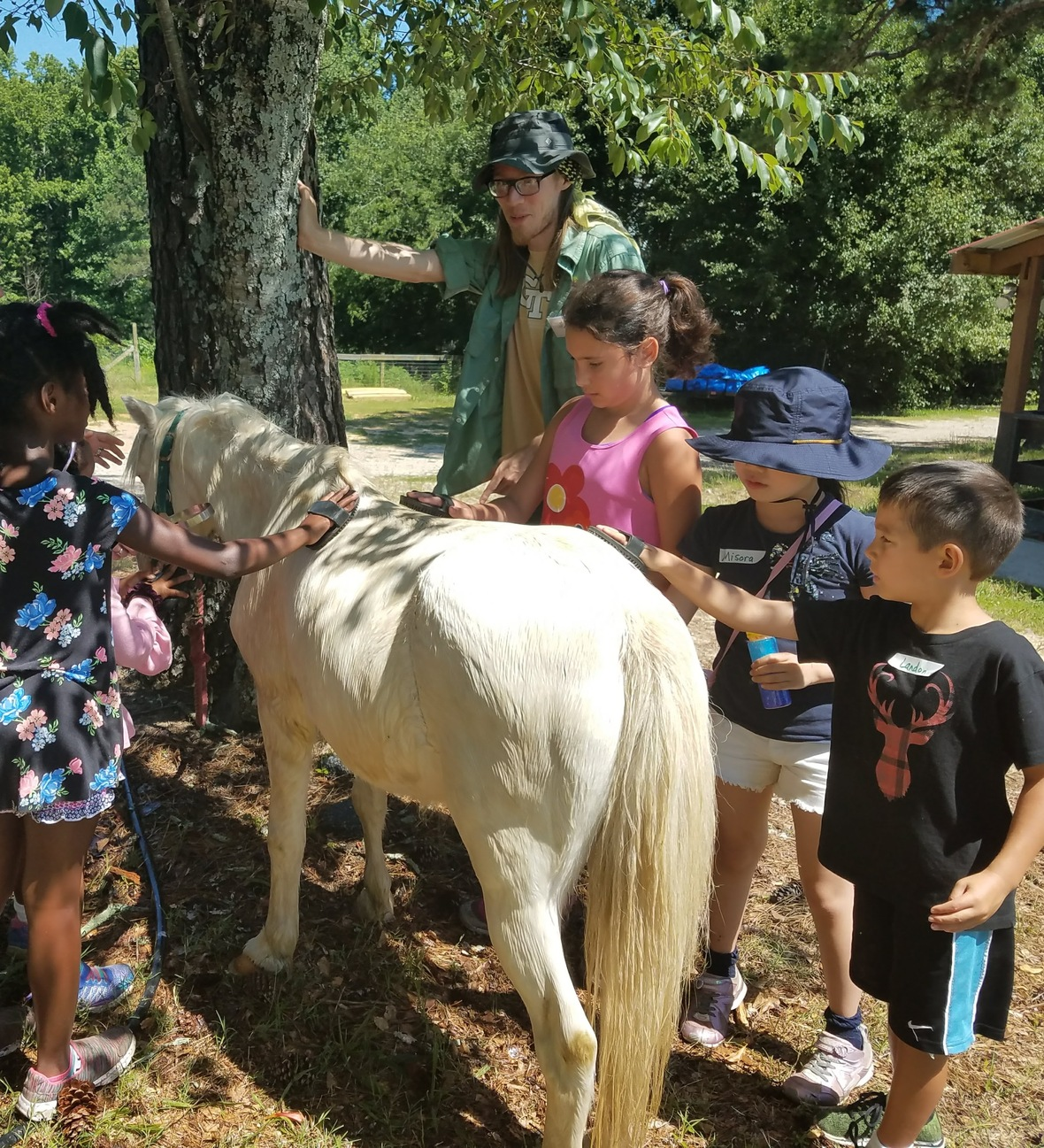It's Time to Register for Summer Camp at Alegre Farm!

Animals, adventures, outdoors, harvesting, cooking, friends, laughs, snowcones, and joy.

What else could a kid ask for?
Well, how about 3 days of excitement!

Get early bird pricing today! Mulitple dates available!

 table div table+table+table+table+table+table+table+table+table+table+table+table+table+table+table+table+table+table+table+table+table+table+table+table+table+table+table+table+table+table+table+table+table+table+table+table+table+table+table+table+table div table{width:100%;padding:0}table div table+table+table+table+table+table+table+table+table+table+table+table+table+table+table+table+table+table+table+table+table+table+table+table+table+table+table+table+table+table+table+table+table+table+table+table+table+table+table+table+table div table img{width:96.23%;padding:0;float:none}table div table+table+table+table+table+table+table+table+table+table+table+table+table+table+table+table+table+table+table+table+table+table+table+table+table+table+table+table+table+table+table+table+table+table+table+table+table+table+table+table+table div table td{width:100%;padding:0 1.88% 18px}/* styles */## Celebrate your team!

 /* styles */ Alegre Farm is the perfect place for your next corporate event! We can help you plan a fun-filled day to give your employees the appreciation they need! Contact us at events@alegrefarm.com to learn more about the different possibilities for your event!
 table div table+table+table+table+table+table+table+table+table+table+table+table+table+table+table+table+table+table+table+table+table+table+table+table+table+table+table+table+table+table+table+table+table+table+table+table+table+table+table+table+table+table+table+table+table div table{width:100%;padding:0}table div table+table+table+table+table+table+table+table+table+table+table+table+table+table+table+table+table+table+table+table+table+table+table+table+table+table+table+table+table+table+table+table+table+table+table+table+table+table+table+table+table+table+table+table+table div table img{width:96.23%;padding:0;float:none}table div table+table+table+table+table+table+table+table+table+table+table+table+table+table+table+table+table+table+table+table+table+table+table+table+table+table+table+table+table+table+table+table+table+table+table+table+table+table+table+table+table+table+table+table+table div table td{width:100%;padding:0 1.88% 18px}/* styles */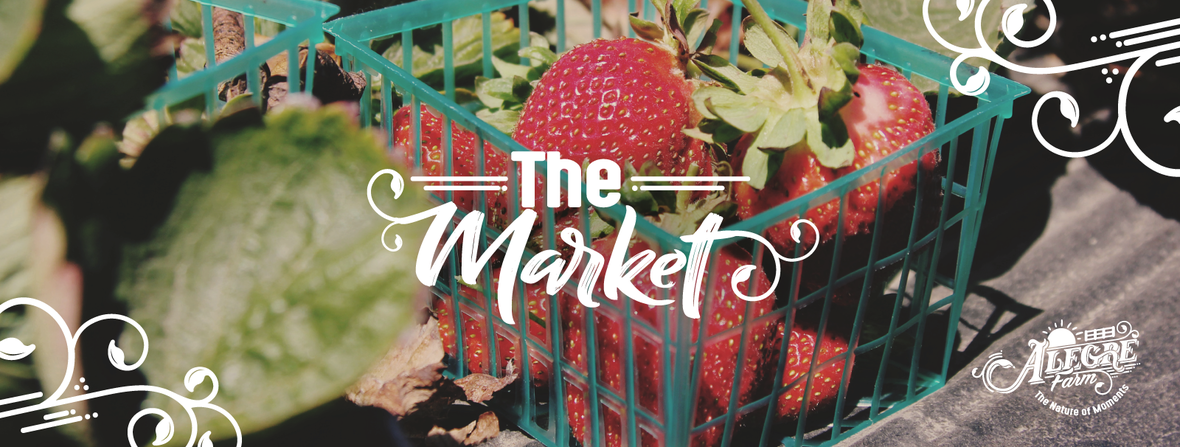## Farmers Market... Fresh, Local, Better

 /* styles */ Support your local economy, Support your local farmer Please consider ordering from our online farmers market.raw milk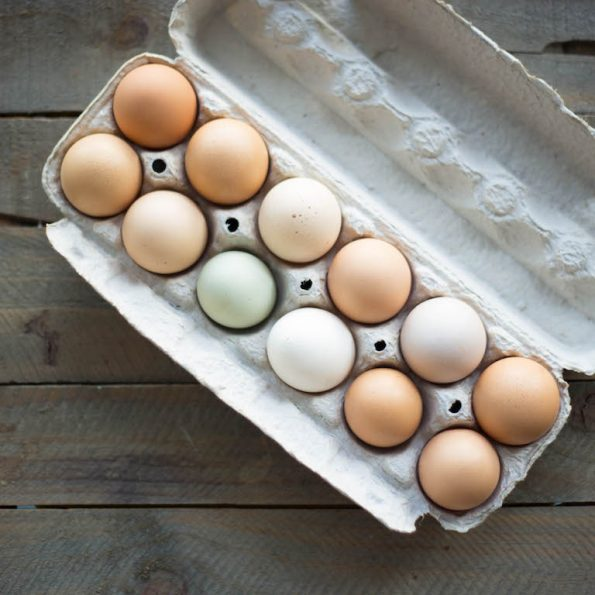fresh eggs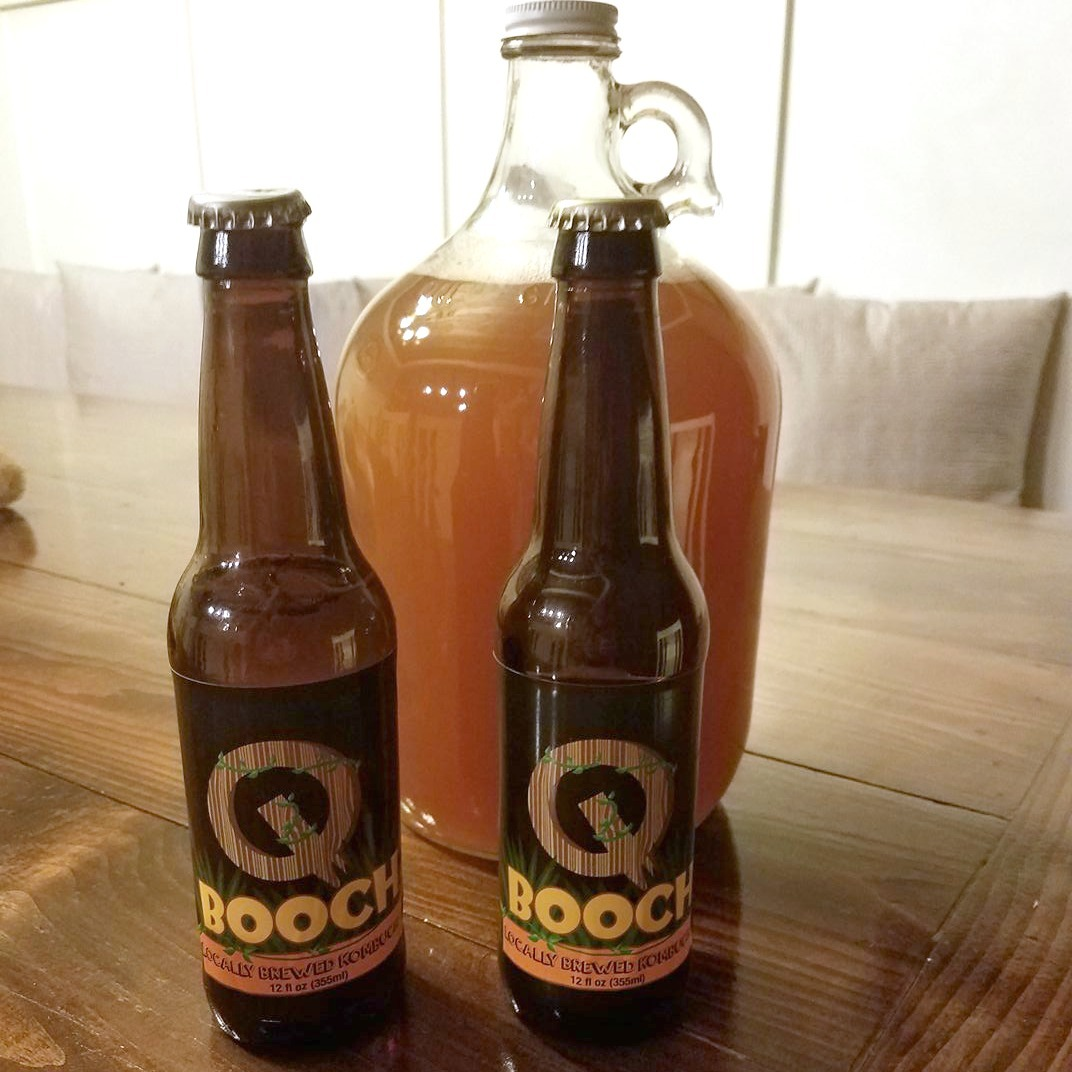kombucha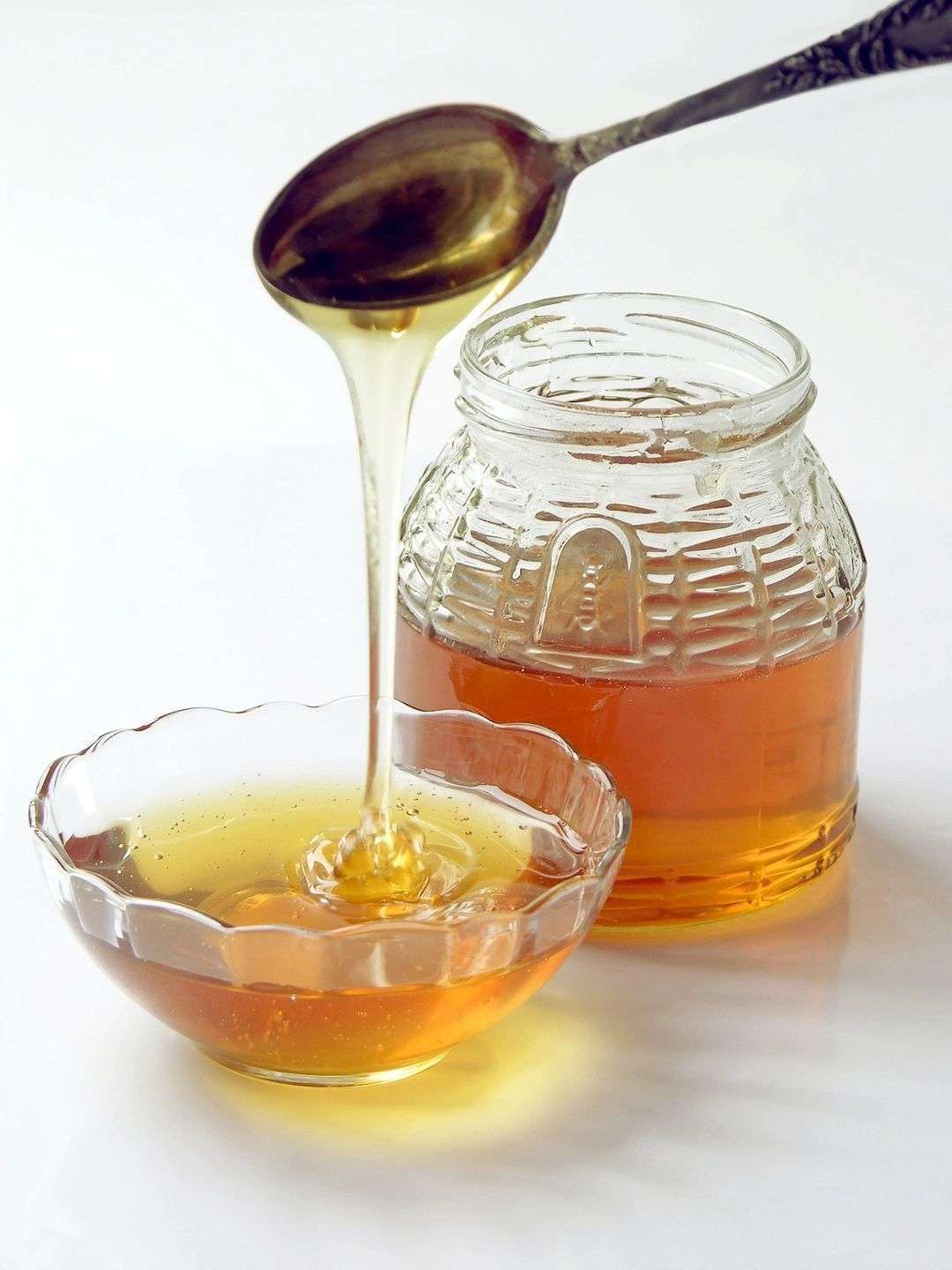raw honey
 table div table+table+table+table+table+table+table+table+table+table+table+table+table+table+table+table+table+table+table+table+table+table+table+table+table+table+table+table+table+table+table+table+table+table+table+table+table+table+table+table+table+table+table+table+table+table+table+table+table+table+table div table{width:100%;padding:0}table div table+table+table+table+table+table+table+table+table+table+table+table+table+table+table+table+table+table+table+table+table+table+table+table+table+table+table+table+table+table+table+table+table+table+table+table+table+table+table+table+table+table+table+table+table+table+table+table+table+table+table div table img{width:96.23%;padding:0;float:none}table div table+table+table+table+table+table+table+table+table+table+table+table+table+table+table+table+table+table+table+table+table+table+table+table+table+table+table+table+table+table+table+table+table+table+table+table+table+table+table+table+table+table+table+table+table+table+table+table+table+table+table div table td{width:100%;padding:0 1.88% 18px}/* styles *//* styles */ Kind regards, Your Team at Alegre FarmLike   Tweet   Pin   +1   in